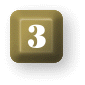## Percentages

Percentage is a term used in arithmetic to denote that a whole quantity divided into 100 equal parts is taken as the standard of measure. Percentage is indicated by the percent sign (%). Thus percent, or %, means a number of parts of one hundred (100). For example, 4% may be written as 4/100 or .04. Notice that 4/100 reduces to 1/25.

 Percents may be added, subtracted, multiplied or divided, just as other specific denominations are treated. 6% + 12% = 18% 8% - 3% = 5% 20% / 5% = 4% 6% x 7% = 42%

To change a percent to a fraction...
...divide the percent quantity by 100 and reduct to lowest terms.
 8% = 8/100 = 2/25 75% = 75/100 = 3/4 80% = 80/100 = 4/5
Fractional Equivalents of Percents

 10% = 1/10 12 1/2% = 1/8 8 1/3% = 1/12 20% = 1/5 25% = 1/4 16 2/3% = 1/6 40% = 2/5 37 1/2% = 3/8 33 1/3% = 1/3 50% = 1/2 62 1/2% = 5/8 66 2/3% = 2/3 60% = 3/5 87 1/2% = 7/8 83 1/3% = 5/6
 To change a percent to a decimal...      ...remove the percent sign and move the decimal point two places to the left. Example: Change 25% to a decimal. 25% = .25     Moving decimal point two places to the left. Example: Change 1.5% to a decimal. 1.5% = .015     To move the decimal point two places to the left, one zero had to be prefixed. To change a decimal to a percent...      ...move the decimal point two places to the right and add a percent sign. Example: Change .24 to a percent. .24 = 24%     Moving decimal point two places to the right and adding the % sign. Example: Change .0043 to a percent. .0043 = .43%     Note that this is less than 1%. Example: Change 2.45 to a percent. 2.45 = 245%     Note that any whole number greater than 1 which designates a percent is more than 100%.

TOP

### Definitions

 The terms commonly used in percentage are: (R) rate (B) base (P) percentage (A) amount (D) difference Rate (R) or rate percent is the fractional part in hundredths that is to be found. Example: 4% of 50 = 2 4%, 1/25 or .04 is the rate. The base (B) is the whole quantity of which some percent is to be found. Example: In 4% of 50 = 2, 50 is the base or whole quantity. The percentage (P) is the result obrained by taking a given hundredth part of the base. Example: In 4% of 50 = 2, 2 is the percentage or the part taken. The amount (A) is the sum of the base and the percentage. Example: In 4% of 50 = 2, 2 the amount is 50 + 2, or 52. The difference (D) is the remainder of the base left when the percentage is subtracted. Example: In 4% of 50 = 2, 50 - 2 or 48 is the difference.

TOP

### The Three Types of Percentage ProblemsFinding a percent
of a number:

given the base and the rate
to find the percentage.
Example 1: Find 14% of \$300.

 \$300 base x .14 rate ______ 1200 300 ______ \$42.00 percentage - Answer
EXPLANATION:
14% is equal to .14.
Multiplying \$300 by .14,
the product is \$42 or 14% of \$300.Finding what percent
one number is of another:

given the base and the percentage
to find the rate.
Example 2: 120 is what percent of 240 ?

 120/240 = 50% - Answer 120 = the percentage 240 = the base 50% = the rate Rate = Percentage / Base    or... E = P / B .
EXPLANATION:
This is another way of saying
what fractional part of 240 is 120.Finding a number
when a percent of that number
is known:

given the rate and the percentage
to find the base.
Example 3: 225 is 25% of what amount ?

 25% = 1/4,   225 / 1/4 = 225 x 4/1 = 900 - Answer 225 = the percentage 20% = the rate 900 = the base Base = Percentage / Rate    or... B = P / R .
EXPLANATION:
This is another way of saying
1/4 of a number equals 225,